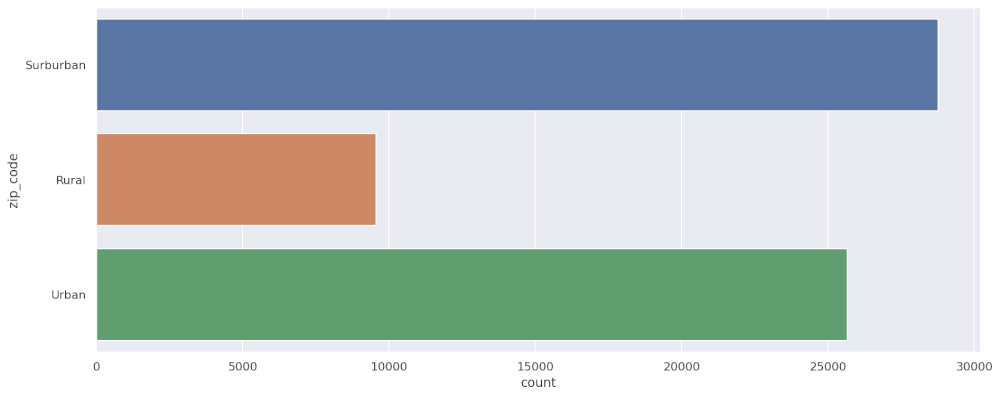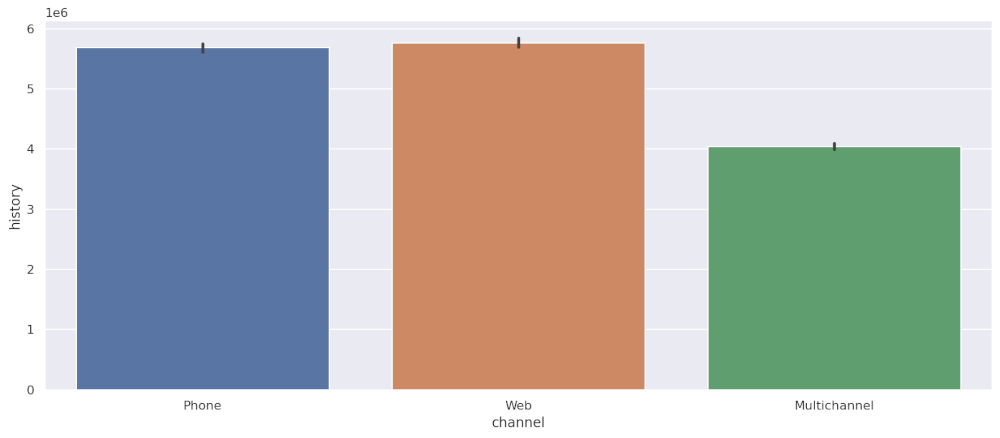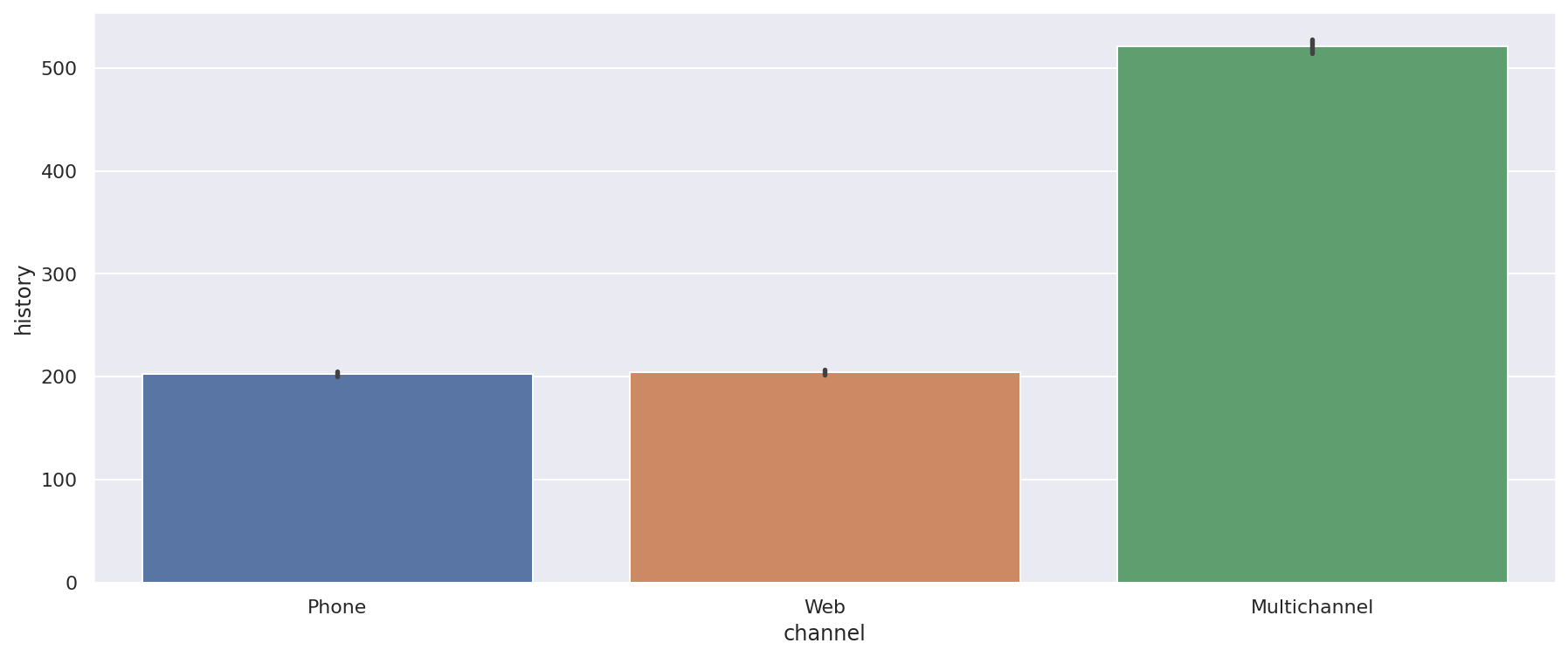Bar. Jacek Dylag, Unsplash.

Barplots or bar charts are probably the most widely used visualisation for displaying and comparing categorical variables. They’re very easy to understand and are quick and easy to generate.

Barplots can be easily constructed solely in Pandas, but using the Seaborn package gives you greater control over the aesthetics of your plots and can make them look more professional than the in-built default design.

Seaborn is a wrapper around the more complex Matplotlib plotting library, which aims to make this very powerful data visualisation package much quicker and easier to use. What would take several lines of code in Matplotlib can be achieved with a quick one-liner command using Seaborn. Here’s how it works.

We only need to use Pandas for loading the data, Numpy for some mathematical stuff, and Seaborn for displaying the charts. However, you may wish to additionally load up the Matplotlib library itself, as this allows you to pass in additional commands. To improve the appearance of our plots, we’ll configure them to use retina mode, so they look sharper, and we’ll tell Seaborn they’re being displayed within a Jupyter notebook at a size of 15 inches by 6 inches by default.

``````import pandas as pd
import numpy as np
import seaborn as sns

%config InlineBackend.figure_format = 'retina'
sns.set_context('notebook')
sns.set(rc={'figure.figsize':(15, 6)})
``````

You can load any dataset you wish, providing it contains the categorical variables you require to display using barplots. I’ve used the Marketing Promotion Campaign Uplift Modelling dataset from Kaggle, which contains several columns of categorical data.

``````df = pd.read_csv('data.csv')
``````
recency history used_discount used_bogo zip_code is_referral channel offer conversion
0 10 142.44 1 0 Surburban 0 Phone Buy One Get One 0
1 6 329.08 1 1 Rural 1 Web No Offer 0
2 7 180.65 0 1 Surburban 1 Web Buy One Get One 0
3 9 675.83 1 0 Rural 1 Web Discount 0
4 2 45.34 1 0 Urban 0 Web Buy One Get One 0

### Count barplots

The simplest barplot to create is a count barplot, as this requires only a single column of data. There’s a special function in Seaborn for plotting count barplots called `countplot()`. This accepts two arguments: `y` (the name of the column to plot) and `data` (the name of the Pandas DataFrame containing the data). Executing this will produce a plot which counts the values present.

``````sns.countplot(y="zip_code", data=df)
``````
``````<matplotlib.axes._subplots.AxesSubplot at 0x7f51c23fa970>
``````### Sum barplots

As the name suggests, a sum barplot or sumplot, shows the sum value of a numeric column mapped against a categorical column. For example, in this example we’re calculating the sum of the `history` column (which contains the total spend for each customer) and are mapping it to the `channel` column (which denotes where the money was taken). That gives us the total spend by channel in a neat chart.

``````sns.barplot(x="channel", y="history", data=df, estimator=sum)
``````
``````<matplotlib.axes._subplots.AxesSubplot at 0x7f51c24c39a0>
``````### Mean barplots

The code above can be easily modified to create a mean barplot. To do this, you simply change the `estimator` argument from `sum` to `np.mean`, which uses the Numpy mean function to calculate the mean spend for each sales channel. As customers can only be multichannel after they’ve purchased in both the Web and Phone channels, it’s the norm for this mean customer spend to be much higher.

``````sns.barplot(x="channel", y="history", data=df, estimator=np.mean)
``````
``````<matplotlib.axes._subplots.AxesSubplot at 0x7f51c24238e0>
``````Matt Clarke, Sunday, March 07, 2021

Matt Clarke Matt is an Ecommerce and Marketing Director who uses data science to help in his work. Matt has a Master's degree in Internet Retailing (plus two other Master's degrees in different fields) and specialises in the technical side of ecommerce and marketing.